## Example Questions

← Previous 1 3 4

### Example Question #51 : Coordinate Geometry

Consider the lines described by the following two equations:

4y = 3x2

3y = 4x2

Find the vertical distance between the two lines at the points where x = 6.

21

44

36

12

48

21

Explanation:

Since the vertical coordinates of each point are given by y, solve each equation for y and plug in 6 for x, as follows:Taking the difference of the resulting -values give the vertical distance between the points (6,27) and (6,48), which is 21.

### Example Question #92 : Coordinate Geometry

For the line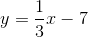Which one of these coordinates can be found on the line?

(9, 5)

(6, 12)

(3, 7)

(6, 5)

(3, 6)

(3, 6)

Explanation:

To test the coordinates, plug the x-coordinate into the line equation and solve for y.

y = 1/3x -7

Test (3,-6)

y = 1/3(3) – 7 = 1 – 7 = -6   YES!

Test (3,7)

y = 1/3(3) – 7 = 1 – 7 = -6  NO

Test (6,-12)

y = 1/3(6) – 7 = 2 – 7 = -5  NO

Test (6,5)

y = 1/3(6) – 7 = 2 – 7 = -5  NO

Test (9,5)

y = 1/3(9) – 7 = 3 – 7 = -4  NO

### Example Question #4 : How To Find Out If A Point Is On A Line With An Equation

Solve the following system of equations:

–2x + 3y = 10

2x + 5y = 6

(–2, 2)

(3, –2)

(2, 2)

(3, 5)

(–2, –2)

(–2, 2)

Explanation:

Since we have –2x and +2x in the equations, it makes sense to add the equations together to give 8y = 16 yielding y = 2.  Then we substitute y = 2 into one of the original equations to get x = –2.  So the solution to the system of equations is (–2, 2)

### Example Question #1 : Other Lines

Which of the following sets of coordinates are on the line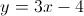?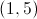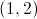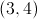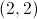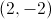Explanation:when plugged in for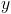andmake the linear equation true, therefore those coordinates fall on that line.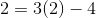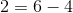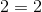Because this equation is true, the point must lie on the line. The other given answer choices do not result in true equalities.

### Example Question #94 : Coordinate Geometry

Which of the following points can be found on the line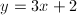?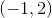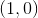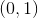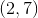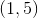Explanation:

We are looking for an ordered pair that makes the given equation true. To solve, plug in the various answer choices to find the true equality.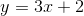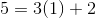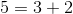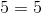Because this equality is true, we can conclude that the pointlies on this line. None of the other given answer options will result in a true equality.

### Example Question #6 : How To Find Out If A Point Is On A Line With An Equation

Which of the following points is on the line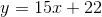?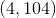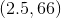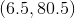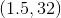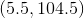Explanation:

The only thing that is necessary to solve this question is to see if a givenvalue will provide you with thevalue paired with it. Among the options provided, onlyworks. This is verified by the following simple substitution: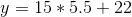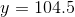### Example Question #1 : How To Find The Slope Of A Line

What is the slope of line 3 = 8y - 4x?

-0.5

2

0.5

-2

0.5

Explanation:

Solve equation for y. y=mx+b, where m is the slope

### Example Question #1 : How To Find The Slope Of A Line

If 2x – 4y = 10, what is the slope of the line?

–0.5

–2

0.5

–5/2

2

0.5

Explanation:

First put the equation into slope-intercept form, solving for y: 2x – 4y = 10 → –4y = –2x + 10 → y = 1/2*x – 5/2. So the slope is 1/2.

### Example Question #111 : Coordinate Geometry

What is the slope of the line with equation 4x – 16y = 24?

1/2

1/4

–1/8

–1/4

1/8

1/4

Explanation:

The equation of a line is:

y = mx + b, where m is the slope

4x – 16y = 24

–16y = –4x + 24

y = (–4x)/(–16) + 24/(–16)

y = (1/4)x – 1.5

Slope = 1/4

### Example Question #1 : How To Find The Slope Of A Line

What is the slope of a line which passes through coordinates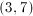and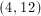?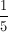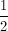Explanation:

Slope is found by dividing the difference in the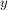-coordinates by the difference in the-coordinates.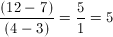← Previous 1 3 4

### All ACT Math Resources